# GMLscripts.com

Discuss and collaborate on GML scriptsYou are not logged in.

## #1 Re: Script Submission » transform_set_HUD » 2008-10-16 06:31:46

Yes and no.  One's the projection transform and the other's the world transform.  They're pretty much the same thing only in different positions.  And I believe that Game Maker views mess with the projection transforms and not the world transforms, so if you change the projection transforms, you can't just clear them to get them back to normal, you have to re-set them to what they were before.  You can certainly use both of them to the same effects, anyway.

Also if you want to get technical, I think d3d_set_projection_ortho nullifies perspective if you have it set, but my method doesn't necessarily (not that that's a particularly good thing for HUDs).

## #2 Script Submission » transform_set_HUD » 2008-09-05 03:04:29

EyeGuy
Replies: 2

A quick, but useful function for drawing a HUD that's not effected by any of the transformations of views (translation, scale, and rotation) without going through the trouble of setting up and optimizing an additional view.

Description:
Sets up a transform which nullifies the scale, translation, and rotation of a given view, making it so that anything you draw afterwards will be drawn unaffected by view.  Calling d3d_transform_set_identity() will change it back to normal.

Expand/*
**  Sample Usage:
**      transform_set_HUD( view_index);
**      // Draw HUD components with position and such as they'll appear on-screen
**      d3d_transform_set_identity();
**
**
**  Arguments:
**      view_index : the index of the view you wish to nullify
**          (in general, this should be view_current)
**
**  Notes:
**      don't forget to call d3d_transform_set_identity(); after you're done drawing
**      all the components of the HUD.  That changes the view back to normal.
**
**  GMLscripts.com
*/
{
d3d_transform_set_scaling( view_wview[argument0]/view_wport[argument0],
view_hview[argument0]/view_hport[argument0],0);
view_hview[argument0]/2 + view_yview[argument0],0);
}

## #3 Re: Game Maker Bugs » draw_line_width_color - confirmed BUG in GM7 » 2008-05-13 01:38:36

Ah, yea, I remember when I was debugging someone else's example, this came up.  Mighty hard to debug something that completely crashes your computer.  I didn't know how he was doing it.

## #4 Re: GML Reference » instance_id[] array » 2008-04-17 14:29:58

Actually what I'm saying is it doesn't get updated in between the alarm event and the normal step event (and thus probably happens right before the begin step event).  My test seems to confirm that.

Edit: of course, this is GM6, but I doubt it changed for GM7.

## #5 Re: GML Reference » Extension Maker v1.2 - GM7 » 2008-04-17 14:18:36

I remember that little annoyance when using the gcc compiler at school.  Interesting to know that the GM7 extension thingy works the same way.

## #6 Re: Script Alumni » gauss - The pseudo-Gaussian » 2008-02-07 17:46:25

The thing is, all the values will be very focused in the middle.  The chances of them getting less than -0.66 or more than 0.66 are very insignificant.  Here's an image of 1,000 blotches created at gauss()*100 pixels away from the center.  The red rectangle shows the range -100 to 100 from the center and you can see there are none anywhere near the edges.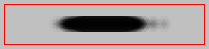I don't know, it really depends on what you want.  If you want a bell-like distribution with higher variance, not likely to get values near the edge, but still possible, and is still between -1 and 1, you could probably get something, but it might not be as quick.  You could use this method divided by like 3 or 4 and force it to re-do itself in the somewhat-unlikely event that it exceeds (-1,1).

## #7 Re: Script Alumni » gauss - The pseudo-Gaussian » 2008-02-07 16:51:53

Those are exactly what I expected.  The variance of X1 - X2 (or "random(1) - random(1)") is 3/18 = 1/6.  Which would mean that the sum of six of them would be approx. 1 = sqrt(6 * 3/18).  And because you divide a distribution with variance approx. 1 by 6, you will get a distribution with variance aprox. (1/6)^2.  More importantly:

Expand// Result: 1.0026576242 (approx. 1)
dsid = ds_list_create();
show_message(string_format(ds_list_variance(dsid),10,10));

What this means is that I think you can get rid of the line "g /= 6;" and you will have a slightly faster function that has approximately the same variance as a the standard normal.

## #8 Re: Script Alumni » gauss - The pseudo-Gaussian » 2008-02-07 11:05:06

Yea, the mean will obviously be 0, but right now the distribution of this pseudo-random function looks like it will have a variance that is significantly different than 1, which would explain the results I got whenever I was testing the two functions a while ago without thinking about how the pseudo-Gauss was working.  It's not a big deal, but if it's going to end up with a normal distribution, it might as well has a variance of 1 (or better yet, have it as an argument like in yourself's exact_gauss script).

According to Wiki, the variance of |X1 - X2| where X1 and X2 are both uniform distributions with the range [0,1) is 1/18.  I should be able to figure out what the variance without the absolute value would be, but it's been a while.  But I think it's 3/18, because X1 - X2 would be a Triangular Distribution with a = -1, b = 0, c = 1, right?

g /= sqrt(6 * 3 / 18);

Wow....  What do you know, that equals 1.  So you don't have to divide by anything at all?

Edit: of course, the 6th iteration of adding won't have EXACTLY a variance of 1, but it should probably be close.  You could even make the number of iterations an argument, but I believe 6 iterations was chosen partially because of the fact that sqrt(6 * Var(X)) = 1.

## #9 Re: Script Alumni » gauss - The pseudo-Gaussian » 2008-02-06 12:18:12

Well, in adition, I believe the distribution uses the Central Limit Theorem, which states that if you take the sum of N identically independentally distributed random variables, subtract the mean from each and divide the whole thing by "sqrt(N) * sigma" (where sigma is the square root of the variance), then as N -> infinity, then the distribution of this will approach a standard Normal (i.e. Gaussian) distribution.

[m]lim{n right infty}{{sum{i=1}{n}{X_{i}} - n mu} / {sqrt{n} sigma}} = W[/m]
(W is a standard normal distribution and each X[i] are each random variables of the same distribution)

Hmm...  should that be line be:

Expand    g /= sqrt(6);

Wait... what's the variance of the triangle distribution "random(1) - random(1)"?  Obviously, the mean is 0, but it seems like the variance probably isn't 1.

## #10 Re: GML Code Help » A question of style: arguments » 2008-02-05 07:09:44

I don't like the idea of changing arguments mid-script.  I know it's equivalent to a call-by-value function in which you are sort of expected to change the value of the argument, but stylistically,  I think "argument0" means the value assigned to an argument and changing the value just seems confusing.

As for using temporary variables for arguments, it all depends on which looks more readable.  I usually just use argument# unless the value is used many times (well, more than once or twice) or it needs to be modified.

If there really is a big difference in adding temporary variables, then for extremely efficient scripts, I could see how you would want to do the faster thing, even changing the argument's value in the script like this.  But for scripts that aren't extremely efficient and likely to be called extreme amounts, then I always say for Game Maker style comes before slight performance difference.

## #11 Re: Off Topic » IGF - Best Freeware Arcade Games 2007 » 2008-01-14 09:03:37

5 out of 20, not bad.  GM certainly seems to be rising in popularity, though obviously the Winter Competition had something to do with it.

I just feel embarrassed that I haven't played a single one of these games.

## #12 Re: Off Topic » Preposterous Code » 2008-01-11 19:23:19

What the... hell.  A silly-straw distribution?

If argument0 is less than argument1, there's an argument0/argument1 chance that it'll return a random number between 0 and argument1.

If argument0 is greater than argument1, then it'll return a random number between 0 and argument0.

It's like a quantum script.  The outcome changed when he measured it.

Ha.

## #13 GML Reference » d3d_transforms » 2008-01-06 04:24:25

EyeGuy
Replies: 2

A supplemental look at d3d_transforms with more detail into how they work and how to use them or a replacement to the old one if you prefer.  If anyone wants to add 3D diagrams for the other rotation transforms, feel free to.

D3D Transformations is a functionality which allows you to geometrically manipulate the points defining the structure of models, 3D shapes, primitives, and even sprites and backgrounds.  Since Game Maker is built off of Direct3D, everything drawn is defined as a set of points to be rendered.  For example, when drawing a sprite, Game Maker defines the 4 corners of the sprite that will be drawn.  Using transforms, you can rotate, scale, and move (translate) the points as much as you want.  And because they are passed through a transform matrix anyway (usually just an identity transform which does nothing to the vertices), you will experience no slowdown other than the slight overhead of defining the transforms.

There are three different types of transformations you can perform:

d3d_transform_set_translation(xt,yt,zt)   Sets the translation over the indicated vector.

Translation: a translation transform will take the vertices used for drawing and simply add whatever coordinates you want to them.  The effect you get will effectively look like you took the object and simply moved it.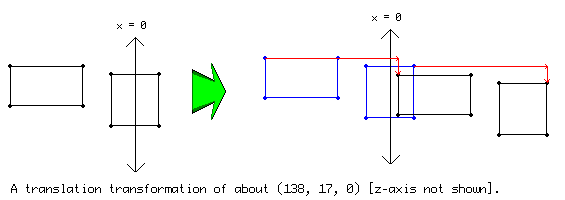d3d_transform_set_scaling(xs,ys,zs)   Sets the transformation to a scaling with the indicated amounts.

Scaling: a scaling transform will squish and expand the vertices about the various axis.  For example, a transform of d3d_transform_set_scaling( 1, 2, 0); will keep the same x coordinates, double the y coordinates and will all have the z coordinate of 0.

NOTE:  For each axis, scaling is done about the line x/y/z = 0.  So objects that are not on this line appear to move in addition to grow.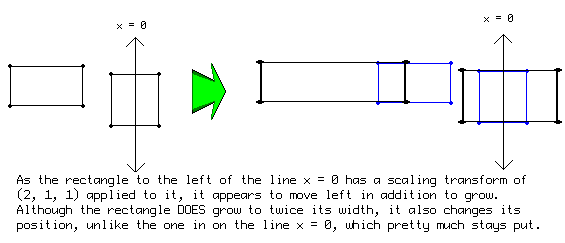d3d_transform_set_rotation_x(angle)   Sets the transformation to a rotation around the x-axis with the indicated amount.

d3d_transform_set_rotation_y(angle)   Sets the transformation to a rotation around the y-axis with the indicated amount.

d3d_transform_set_rotation_z(angle)   Sets the transformation to a rotation around the z-axis with the indicated amount.

d3d_transform_set_rotation_axis(xa,ya,za,angle)   Sets the transformation to a rotation around the axis indicated by the vector with the indicated amount. d3d_transform_add_translation(xt,yt,zt)

d3d_transform_add_rotation_x(angle)   Adds a transformation to a rotation around the x-axis with the indicated amount.

d3d_transform_add_rotation_y(angle)   Adds a transformation to a rotation around the y-axis with the indicated amount.

d3d_transform_add_rotation_z(angle)   Adds a transformation to a rotation around the z-axis with the indicated amount.

d3d_transform_add_rotation_axis(xa,ya,za,angle)   Adds a transformation to a rotation around the axis indicated by the vector with the indicated amount. d3d_transform_add_translation(xt,yt,zt)

Rotation: there are three different type of rotation transforms, but the principle is the same in all three.  The coordinates are rotated about a given axis.  Imagine taking a pencil attached to a piece of paper or a 3D object like an apple.  As you twist the pencil (assuming it's sufficiently stuck in the object), you will see the effect that rotating about the axis the pencil represents will give you.  For example, if you want to rotate 2D coordinates the way we normally do, you want to (perhaps counter-intuitively) rotate about the z-axis which can be thought as pointing towards you in a 2D game.

NOTE: Like in scaling, rotation is done about the point (0,0,0).  That means objects which are not on this point will appear to move as they rotate.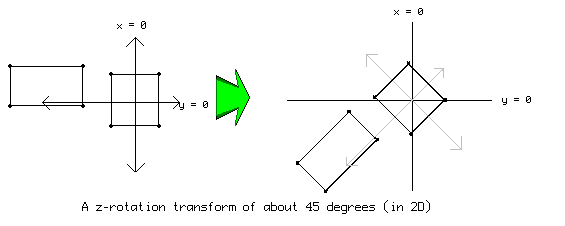The x and y rotations are similar to the z rotation, but they have less meaning in 2D (they'll just be trigonometric scaling assuming perspective is turned off).  Playing around with these in 3D, it should be fairly obvious how they work.

The last rotation transform might warrant a bit more explanation.  In the axis rotation, you define the axis you want them to rotate arround in addition to how many degrees you want them to rotate around that axis.  Imagine a globe revolving arround its axis.  The axis it's being rotated around is define as the vertex from the center of the globe to the point outside which the globe is affixed to whatever it rotates inside.

d3d_transform_set_identity()   Sets the transformation to the identity (no transformation).

Transforms effect everything that is drawn after the transform is set, including sprites/backgrounds and 2D shapes in addition to anything 3D.  So after you draw everything you want drawn, remember to call d3d_transform_set_identity(); so that everything else is drawn normally.  For example, drawing a sprite at 0,0 rotated 45 degrees then a sprite at 100,0 not rotated at all:

Expand{
d3d_transform_set_rotation_z( 45);
draw_sprite(spr,0,0,0);
d3d_transform_set_identity();
draw_sprite(spr2,0,100,0);
}

The difference between the d3d_transform_add_*** and the d3d_transform_set_*** is that d3d_transform_set_*** will clear all the transforms before it, where the d3d_transform_add_** "adds" the new transform to the old ones.  What does it mean to add a transform onto an old one?  Well, imagine if you could only apply one transform to whatever shape you wanted.  This would be mighty inconvenient because anything you wanted rotated would either have to appear at (0,0,0) or would be some crazy place you can't predict.  So what you do is you apply a rotation to the object THEN a translation to the object so that it will be rotated about the point (0,0,0) and then will be moved to the correct place.

Expand{
d3d_transform_set_identity();
draw_sprite(spr,0,0,0);
d3d_transform_set_identity();
}

How on earth can you keep track of what cascading transforms will accomplish and how to logically justify what happens to them?  Well, you have to start with the end.  That is to say, you start by imagining (or drawing) where the points that are drawn in the draw statement after all the transforms.  Then, one-by-one, apply the next transform you defined to the points you end up with from the previous transform.

For example, in the following code:

Expand{
d3d_transform_set_identity();
draw_triangle(0,0,0,50,50,50,1);
d3d_transform_set_identity();
}

You start with the coordinates of the triangle and then one-by-one apply the transforms: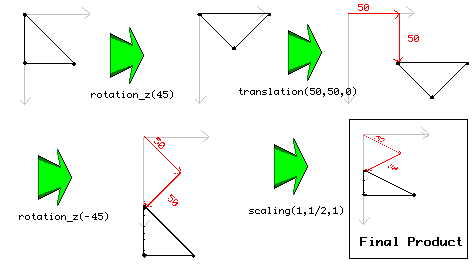As you can see, transforms can be used both in 2D and in 3D.  Even though you can think of them as a series of actions on each and every vertex, the fact is all these actions are stored on a single fixed-size matrix (through matrix multiplication) and so you shouldn't expect lag from drawing an object after you applied thousands of transforms to it.  The following functions can help you build such a stack without having to have it always applied to everything drawn.  They create ways of storing the current transformation so you can get rid of it and use it later.  They also work great for hierarchical structures.

d3d_transform_stack_clear()   Clears the stack of transformations.

d3d_transform_stack_empty()   Returns whether the transformation stack is empty.

d3d_transform_stack_push()   Pushes the current transformation on the stack. Returns whether there was room on the stack to push it there (if you forget popping transformation you at some moment will run out of room on the stack).

d3d_transform_stack_pop()   Pops the top transformation from the stack and makes it the current one. Returns whether there was a transformation on the stack.

d3d_transform_stack_top()   Makes the top transformation the current one, but does not remove it from the stack. Returns whether there was a transformation on the stack.

d3d_transform_stack_discard()   Removes the top transformation from the stack but does not make it the current one. Returns whether there was a transformation on the stack.

Make sure you don't create an infinitely-growing stack, or create a stack underflow by popping a stack that's empty.

One use of the d3d_transform_stack functions that makes use of this ever-growing series of transforms things is an easy way to get "perfect" ball rotation.  This only works for one ball at a time, but in the create event, push a normal identity transform and then in the draw event, apply an axis rotation to the already-rotated vertices:

Expand{
d3d_transform_stack_pop();
d3d_transform_add_rotation_axis( -vy, vx, 0, dist * 2.3);
d3d_transform_stack_push();

d3d_draw_ellipsoid( - 0.5, - 0.5, - 0.5, 0.5, 0.5, 0.5, 0, 1, 1, 8);
d3d_transform_set_identity();
}

The transform stack will memorize the previous rotation of the ball so that it's a simple matter of applying the new rotation and then memorizing it.  Remember, you have to make sure the translation is outside of that stack because when you rotate an object that is translated so that it is not centered at the point (0,0,0), crazy things happen.

Well, that's my knowledge of transformed, I hope this helps you understand this powerful tool built into the engine GM's built off of.  Here's hoping Game Maker has more transform functionallity in the future.  In particular better ways of storing more matrix transforms and ways of applying the various transforms in the other direction in the future (which'd make heirarchical constructions a whole lot easier).

-EyeGuy

## #14 Script Alumni » draw_sprite_tiled_area[_ext] - slight improvement » 2007-11-11 02:01:52

EyeGuy
Replies: 2

I don't know if this is the proper place since it's my own script and the changes are somewhat minor and the scripts kinda out-dated since surfaces have been introduced, but this has always been a real old script of mine that's I thought I could improve/understand better.  Also, I got xscale/yscale working on the _ext version, though scaled down tiled images don't look the smoothest.

Expand/*
**  Usage:
**      draw_sprite_tiled_area(sprite,subimg,x,y,x1,y2,x2,y2)
**
**  Arguments:
**      sprite  the sprite to be drawn
**      subimg  the sub image of the sprite to be drawn
**      x,y     the offset of the tiled area, as defined by a point
**              the sprite will/would be drawn
**      x1,y1   top left corner of the rectangle defining the area
**      x2,y2   bottom right corner of the area
**
**  Notes:
**      (x,y) doesn't have to be in the area, but if it is, then some
**          drawn sprite will have it's origin at this point
**
**  GMLscripts.com
*/
{
var sprite,subimg,xx,yy,x1,y1,x2,y2;
sprite = argument0;
subimg = argument1;
xx = argument2 + sprite_get_xoffset(sprite);
yy = argument3 + sprite_get_yoffset(sprite);
x1 = min(argument4,argument6);
y1 = min(argument5,argument7);
x2 = max(argument4,argument6);
y2 = max(argument5,argument7);

var sw,sh,i,j,jj,left,top,width,height,X,Y;
sw = sprite_get_width(sprite);
sh = sprite_get_height(sprite);

i = x1 - (((x1 - xx) mod sw + sw) mod sw);
j = y1 - (((y1 - yy) mod sh + sh) mod sh);
jj = j;

for(i=i; i<=x2; i+=sw) {
for(j=j; j<=y2; j+=sh) {
if(i <= x1) left = x1-i;
else left = 0;
X = i+left;

if(j <= y1) top = y1-j;
else top = 0;
Y = j+top;

if(x2 <= i+sw) width = 1+x2-i-left;
else width = sw-left;

if(y2 <= j+sh) height = 1+y2-j-top;
else height = sh-top;

draw_sprite_part(sprite,subimg,left,top,width,height,X,Y);
}
j = jj;
}
}

Changes:
-automatically detects the lowest and highest points so you don't have to worry about it
-adds in the offset of the sprite to the x/y offset because draw_sprite_part ignores the offset
-the bulky i = ... and j = ... formula is replaced by one very similar to the angle_distance formula which is smaller and faster
-some redundant math in the if(...) width/height = .... lines gotten rid of

Expand/*
**  Usage:
**      draw_sprite_tiled_area_ext(sprite,subimg,x,y,x1,y2,x2,y2,xscale,yscale,rotation,color,alpha)
**
**  Arguments:
**      sprite  the sprite to be drawn
**      subimg  the sub image of the sprite to be drawn
**      x,y     the offset of the tiled area, as defined by a point
**              the sprite will/would be drawn
**      x1,y1   top left corner of the rectangle defining the area
**      x2,y2   bottom right corner of the area
**      xs,ys   the xscale and yscale
**      color   the color mask that you want to blend the sprite with
**      alpha   the alpha value to draw it at
**
**  Notes:
**      (x,y) doesn't have to be in the area, but if it is, then some
**      drawn sprite will have it's origin at this point
**
**  GMLscripts.com
*/
{
var sprite,subimg,xx,yy,x1,y1,x2,y2,xs,ys;
sprite = argument0;
subimg = argument1;
xx = argument2 + sprite_get_xoffset(sprite);
yy = argument3 + sprite_get_yoffset(sprite);
x1 = min(argument4,argument6);
y1 = min(argument5,argument7);
x2 = max(argument4,argument6);
y2 = max(argument5,argument7);
xs = argument8;
ys = argument9;
if(xs == 0) xs = 1;
if(ys == 0) ys = 1;

var sw,sh,i,j,jj,left,top,width,height,X,Y;
sw = sprite_get_width(sprite)*xs;
sh = sprite_get_height(sprite)*ys;

i = x1 - (((x1 - xx) mod sw + sw) mod sw);
j = y1 - (((y1 - yy) mod sh + sh) mod sh);
jj = j;

for(i=i; i<=x2; i+=sw) {
for(j=j ;j<=y2; j+=sh) {

if(i <= x1) left = (x1-i);
else left = 0;
X = i+left;

if(j <= y1)top = (y1-j);
else top = 0;
Y = j+top;

if(x2 <= i+sw) width = 1+x2-i-left;
else width = sw-left;

if(y2 <= j+sh) height = 1+y2-j-top;
else height = sh-top;

draw_sprite_part_ext(sprite,subimg,left/xs,top/ys,width/xs,height/ys,X,Y,xs,ys,argument10,argument11);
}
j = jj;
}
}

Changes:
-same as above
-slight changes to add in xscale and yscale

## #15 Re: Script News & Announcements » Mathematical Expressions » 2007-10-19 21:08:57

Seems pretty robust.  Not a lot of the specific notations, but a lot of that you can create out of what they do have.  As mentioned, it's not nearly as pretty as LaTeX, as I noticed whenever I created a bracketted set with subscripts.  If installing LaTeX is really too much of a pain, this system really does work probably for everything it'll ever be used for.

Testing (ignore whatever it is it ended up saying):
1)[m]{A_{n}}=delim{lbrace}{a_{0}} , cdots,{a_{n}}{rbrace} backslash delim{lbrace}{a_{n+1}} , cdots,{a_{infty}}{rbrace}[/m]
[m]{a_{n}}in{A_{n}} forall {n} doubleleftright {a_{i}}<>{a_{j}} forall {j}<>{i}[/m]

2)[m]{overline{lim}}under{{n}right{+infty}}{a_{n}} = {c},~{c}in bbR~doubleright lim{{n}right{+infty}}{a_{n}} <= {c}[/m] (if the limit exists)

3)[m]delim{[}{matrix{3}{3}{1 0 0 0 1 0 0 0 1}}{]}*delim{A} = {A}[/m]

Seems to get the point across.  Not the best spacing, though maybe that's partially my responsibility.  Not sure why my first commas aren't showing up, though.

## #16 Re: Community » Introductions » 2007-10-18 15:56:26

EyeGuy (along with a few other aliases).  Found Game Maker through Google back in 2003 or whenever GM 4.1 was.  Had lots and lots of trouble with it until I realized it came with its own scripting language (or whatever the technical term for what GML is).  Having already had a class or two worth of C++ in high school, using GML came naturally and I proceeded to make dozens of dozens of platformer engines, none of which ever amounted to anything.  Registered at the GMC a good deal after finding Game Maker and answer questions in the AQ&A from time to time.  Usually answering the hardest questions I can find.